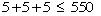Subject: equation how make true To whome it may concern, I am in algebra and my teacher gave us an equation that was not true. she told us that we could only use one line segment(it can't bend turn has to be straight) to make the equation true. here is the equation: 5+5+5=550. i have not figured it out but have tried many things and believe it is not mathmatical but cross a # or sign out.also i forgot you can't put a slash mark through the equals sign. if u have any questions or if you figure it out please tell me i nees the extra credit. thankyou, Tom K-12 Student Hi Tom, How aboutCheers, Penny Tom:Here is a suggestion from Leeanne I will give you a hint: the RHS of the equation and one of the plus signs must remain unchanged. Now consider what the rest of the equation must be in order for it to be true. Go to Math Central